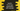# Swift 4 for-in loop Tutorial with Example## for-in loop in Swift-4 :

For-in loop is used to iterate over sequence like array, dictionary, string etc. In this example, I will show you use of for-in loop with different examples.Drop a comment if you have any queries.

### For-in loop to iterate over an array :

Using for-in loop, we can iterate through each elements of an array like below :

``````var daysArray = ["SunDay","MonDay","TuesDay","WednesDay","ThursDay","FriDay","SaturDay"]

for day in daysArray{
print (day)
}
``````

Output :

``````SunDay
MonDay
TuesDay
WednesDay
ThursDay
FriDay
SaturDay
``````

### Iterating over a dictionary using for-in loop :

Dictionary iteration is same as array using for-in loop. We can iterate and access each key-value pairs of a dictionary. But since the items of a dictionary are not ordered, it doesnot gurantee the order in which they will be retrieved.

``````var daysDictionary = [1 : "SunDay",2 : "MonDay",3 : "TuesDay",4 : "WednesDay",5 : "ThursDay",6 : "FriDay",7 : "SaturDay"]

for (id,day) in daysDictionary{
print ("\(id) , \(day)")
}
``````

Output :

``````2 , MonDay
4 , WednesDay
5 , ThursDay
6 , FriDay
7 , SaturDay
3 , TuesDay
1 , SunDay
``````

### With Numeric ranges - Print Multiplication table :

for-in loop can be used with numeric ranges directly . 1…n means it will take values from 1 to n (including n). Following example will print the multiplication table for 9 :

``````for count in 1...10 {
print ("9 * \(count) = \(count * 9)")
}
``````

Output :

``````9 * 1 = 9
9 * 2 = 18
9 * 3 = 27
9 * 4 = 36
9 * 5 = 45
9 * 6 = 54
9 * 7 = 63
9 * 8 = 72
9 * 9 = 81
9 * 10 = 90
``````

In the above example, we are storing the current numeric value in a variable count. If the value is not using inside the loop, we can simply write ’_’ instead of any variable name. Below example is to find the factorial of a number and it is illustrating this approach.

``````var fact = 1
var number = 10

var counter = number

for _ in 2...number{
fact *= counter
counter = counter - 1
}
print ("factorial of \(number) is \(fact)")
``````

Output :

``````factorial of 10 is 3628800
``````

### Half open range operation in for-in loop :

_Half open range operator _ (a..<b) means that it will run from a to b but will not include b.

``````for i in 1..<10 {
print ("i = \(i)")
}
``````

In the above example, it will run from i=1 to i=9 . output is :

``````i = 1
i = 2
i = 3
i = 4
i = 5
i = 6
i = 7
i = 8
i = 9
``````

### One-sided ranges operation in for-in loop (Swift-4) :

Closed range operator , [a…] starts from ‘a’ and it will continue as far as possible. If we use it on an array, it will continue from ‘a’ to the end of the array .

``````var daysArray = ["SunDay","MonDay","TuesDay","WednesDay","ThursDay","FriDay","SaturDay"]

for day in daysArray[3...]{
print (day)
}
``````

Output :

``````WednesDay
ThursDay
FriDay
SaturDay
``````

Similarly for […a] and [..<a] :

``````var daysArray = ["SunDay","MonDay","TuesDay","WednesDay","ThursDay","FriDay","SaturDay"]

for day in daysArray[...3]{
print (day)
}
print ("---------")

for day in daysArray[..<3]{
print (day)
}
``````

Output :

``````SunDay
MonDay
TuesDay
WednesDay
---------
SunDay
MonDay
TuesDay
``````

### Iterate with an interval using for in loop :

We can also iterate with an interval using stride(from,to,by) method. ‘from’ means the starting point, ‘to’ means the ending point ( but it will not be considered )and ‘by’ means interval between each step. Example :

``````for number in stride(from: 0, to: 10, by: 2) {
print (number)
}
``````

Output :

``````0
2
4
6
8
``````

In the above example,10 is not included. If you want to include it , you can use stride(from,through,by) method. So, if ‘from’ is 0 and ‘through’ is 10 , 0 to 10 : all numbers will be considered. If we use this for the same above example :

``````for number in stride(from: 0, through: 10, by: 2) {
print (number)
}
``````

Output :

``````0
2
4
6
8
10
``````

It includes 10.